# Class 7 Maths NCERT Solutions for Chapter 2 Fractions and Decimals EX – 2.2

## Fractions and Decimals

Question 1.
Which of the drawings (a) to (d) show :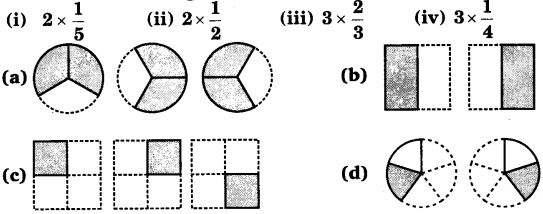Solution:
(i) → (d)
(ii) → (b)
(iii) → (a)
(iv) → (c)

Question 2.
Some pictures (a) to (c) are given below. Tell which of them show :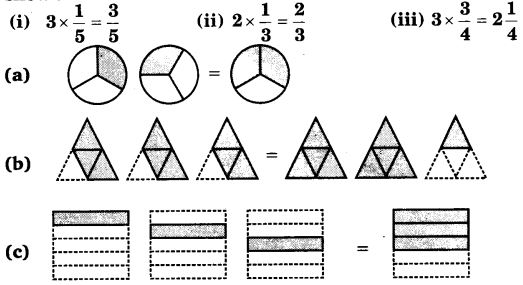Solution:
(i)  → (c)
(ii) → (a)
(iii) → (b)

Question 3.
Multiply and reduce to lowest form and convert into a mixed fraction.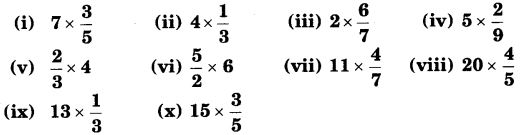Solution:

Question 4.
(i)$\frac { 1 }{ 2 }$ of the circles in box (a)
(ii)$\frac { 2 }{ 3 }$ of the triangles in box (b)
(iii)$\frac { 3 }{ 5 }$ of the squares in box (c).Solution:

Question 5.
Find :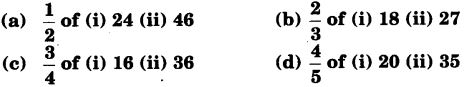Solution:

Question 6.
Multiply and express as a mixed fraction :Solution:

Question 7.
Find :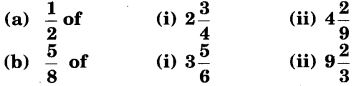Solution:

Question 8.
Vidya and Pratap went for a picnic. Their mother gave them a water bottle that contained 5 liters of water. Vidya consumed$\frac { 2 }{ 5 }$ of the water. Pratap consumed the remaining water.
(i)
How much water did Vidya drink?
(ii) What fraction of the total quantity of water did Pratap drink ?

Solution:

#### Class 7 Maths NCERT Solutions for Chapter 2 Fractions and Decimals EX – 2.3

Lorem ipsum dolor sit amet, consectetur adipiscing elit. Phasellus cursus rutrum est nec suscipit. Ut et ultrices nisi. Vivamus id nisl ligula. Nulla sed iaculis ipsum.

Company Name